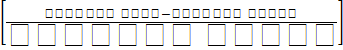The rate of Return can be calculated by the formula:-

The price of Return=Current Rate-Initial Value

Initial Value=x 100